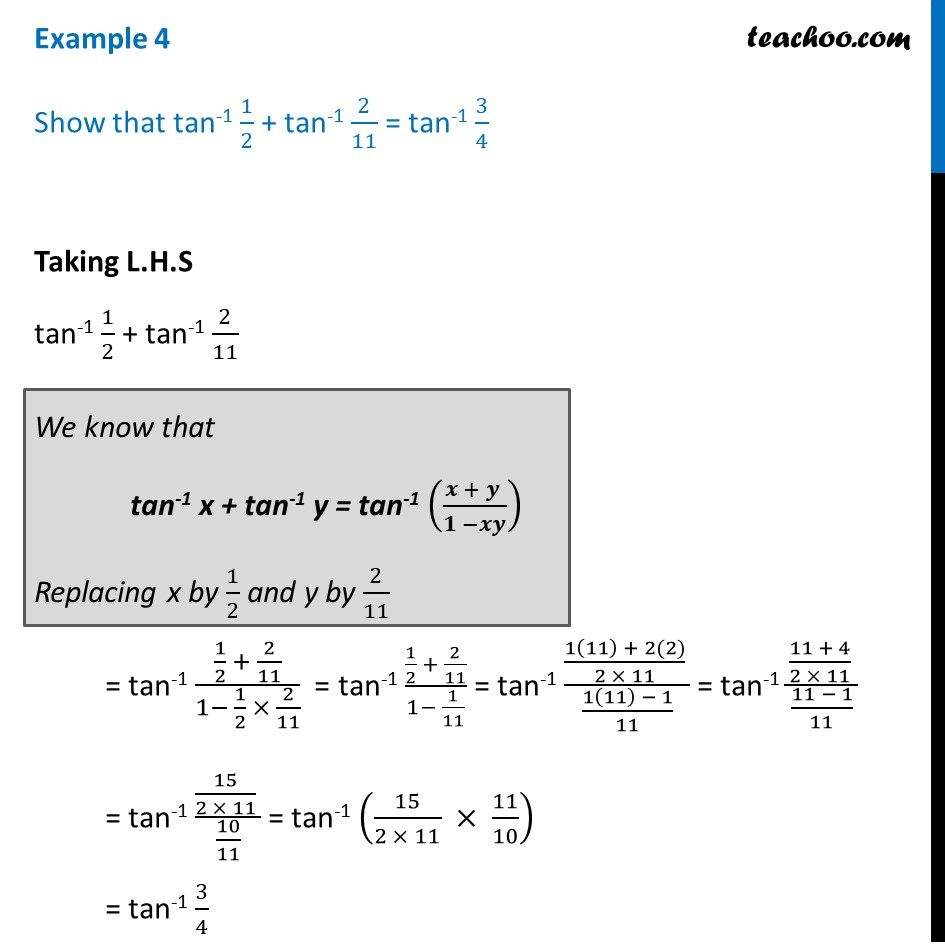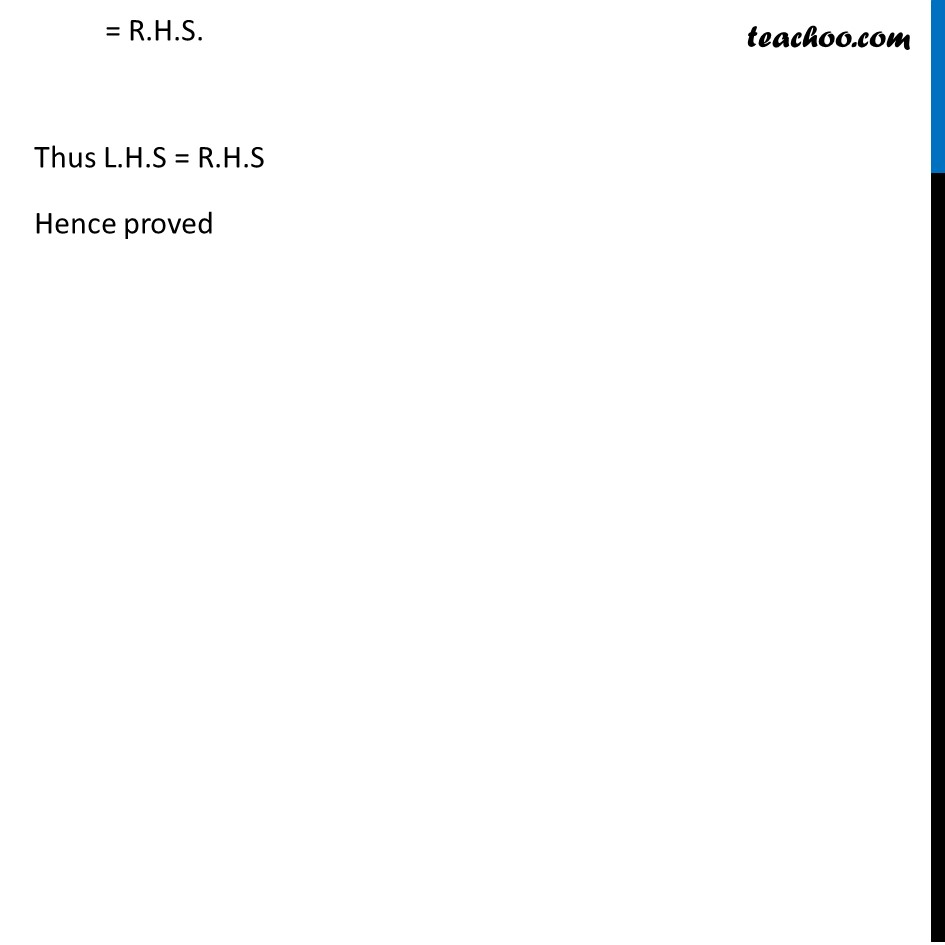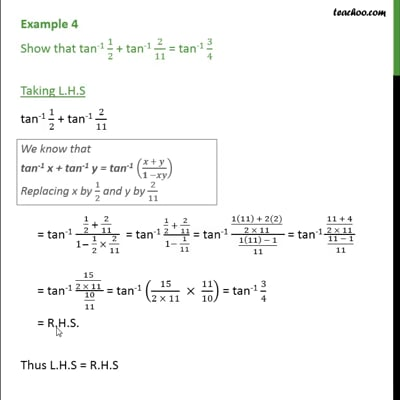Examples

Chapter 2 Class 12 Inverse Trigonometric Functions
Serial order wiseThis video is only available for Teachoo black users

Solve all your doubts with Teachoo Black (new monthly pack available now!)

### Transcript

Example 4 Show that tan-1 1/2 + tan-1 2/11 = tan-1 3/4 Taking L.H.S tan-1 1/2 + tan-1 2/11 = tan-1 (1/2 + 2/11)/(1− 1/2 × 2/11) = tan-1 (1/2 + 2/11)/(1− 1/11) = tan-1 ((1(11) + 2(2))/(2 × 11) )/((1(11) − 1)/11) = tan-1 ((11 + 4)/(2 × 11) )/((11 − 1)/11) = tan-1 (15/(2 × 11) )/(10/11) = tan-1 (15/(2 × 11) × 11/10) = tan-1 3/4 We know that tan-1 x + tan-1 y = tan-1 ((𝒙 + 𝒚 )/(𝟏 −𝒙𝒚)) Replacing x by 1/2 and y by 2/11 = R.H.S. Thus L.H.S = R.H.S Hence proved